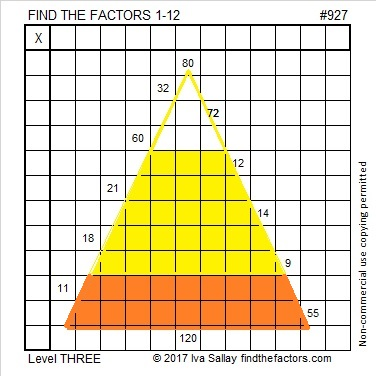# 927 Candy Corn

Candy corn is a traditional Halloween candy.

Figure out what number goes in the top cell of the first column of this level three candy corn puzzle, and work your way down the first column, cell by cell, to make this puzzle a treat to complete.Print the puzzles or type the solution on this excel file: 12 factors 923-931

Fibonacci numbers begin with 1, 1, with the rest of the numbers in the sequence being the sum of the previous two.

Tribonacci numbers begin with 0, 0, 1 with the rest of the numbers in the sequence being the sum of the previous THREE.

The first 15 tribonacci numbers are 0, 0, 1, 1, 2, 4, 7, 13, 24, 44, 81, 149, 274, 504, 927. Thank you, OEIS.org for that fun fact.

• 927 is a composite number.
• Prime factorization: 927 = 3 × 3 × 103, which can be written 927 = 3² × 103
• The exponents in the prime factorization are 2 and 1. Adding one to each and multiplying we get (2 + 1)(1 + 1) = 3 × 2  = 6. Therefore 927 has exactly 6 factors.
• Factors of 927: 1, 3, 9, 103, 309, 927
• Factor pairs: 927 = 1 × 927, 3 × 309, or 9 × 103
• Taking the factor pair with the largest square number factor, we get √927 = (√9)(√103) = 3√103 ≈ 30.44667